# Motion and Energy Motion position motion 1 A

• Slides: 16Motion and Energy Motionposition, motion 1. A ________ is the location of an object. A change in the position of an object over time is ______.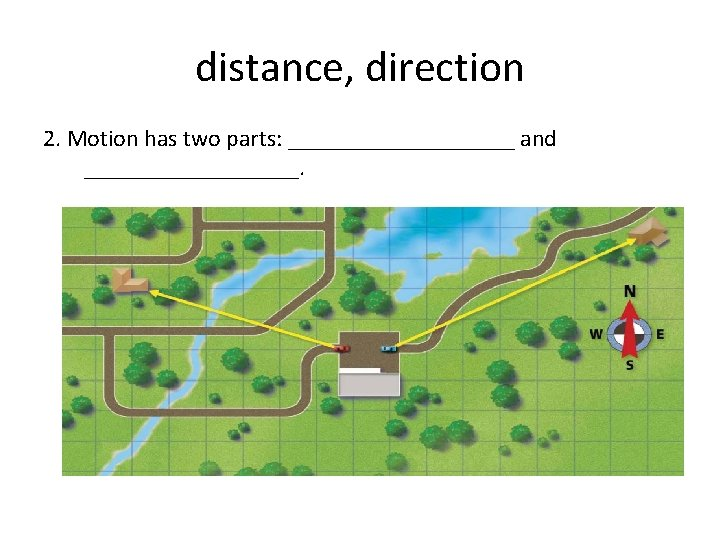distance, direction 2. Motion has two parts: __________ and _________.meters (m), kilometers (km), feet (ft), or miles (mi) 3. Distance can be measured in _______________( ), ______________ ( ), or ________________( ).compass , protractor 4. To measure direction, you can use a ___________ or a __________ and units of degrees.frame of reference 5. Positions and motions only make sense if you have a __________. A frame of reference is a group of objects from which you measure a position or the motion. Almost anything can be a frame of reference and frames of reference can move.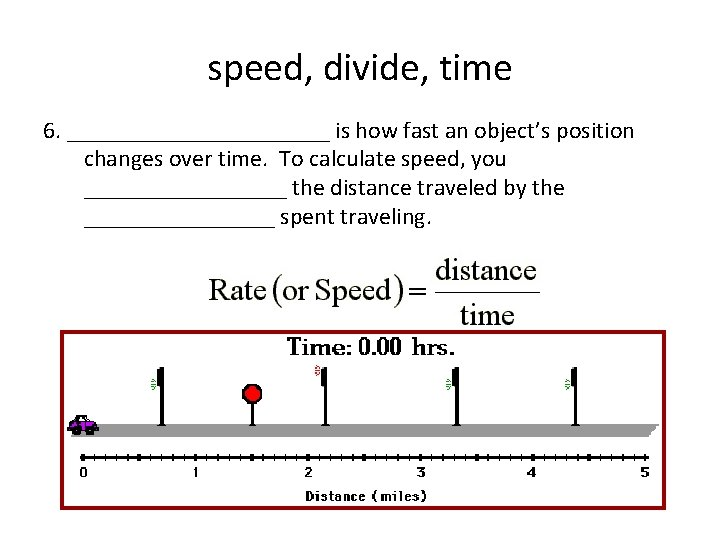speed, divide, time 6. ___________ is how fast an object’s position changes over time. To calculate speed, you _________ the distance traveled by the ________ spent traveling.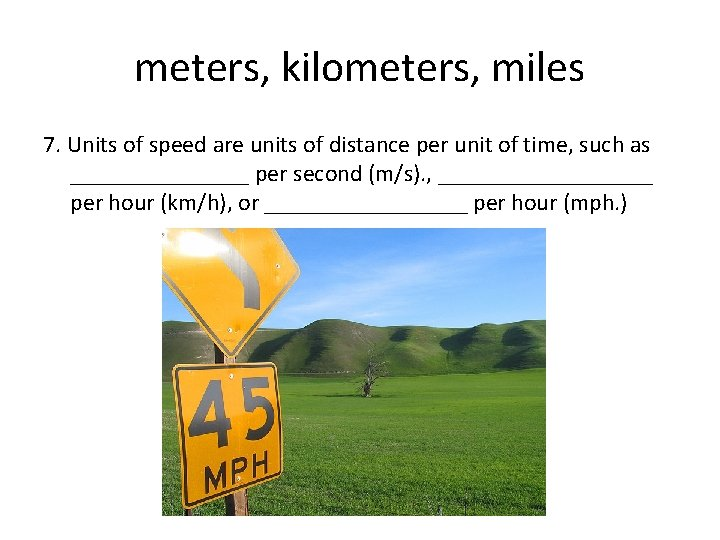meters, kilometers, miles 7. Units of speed are units of distance per unit of time, such as ________ per second (m/s). , _________ per hour (km/h), or _________ per hour (mph. )velocity, speed, direction 8. __________ is the measurement that combines both _______ and _________ of a moving object.acceleration 9. __________ is the change in velocity over time of an object. Since units of velocity include speed and direction, if either of those changes, the object is considered to be accelerating.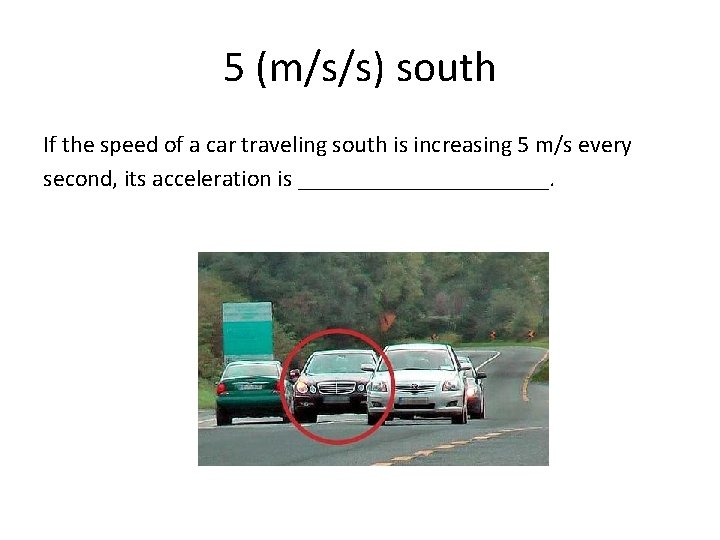5 (m/s/s) south If the speed of a car traveling south is increasing 5 m/s every second, its acceleration is ___________.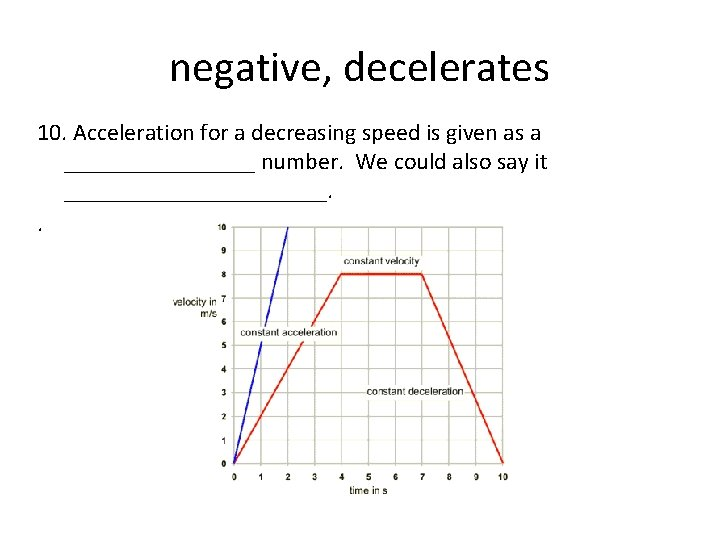negative, decelerates 10. Acceleration for a decreasing speed is given as a ________ number. We could also say it ___________. .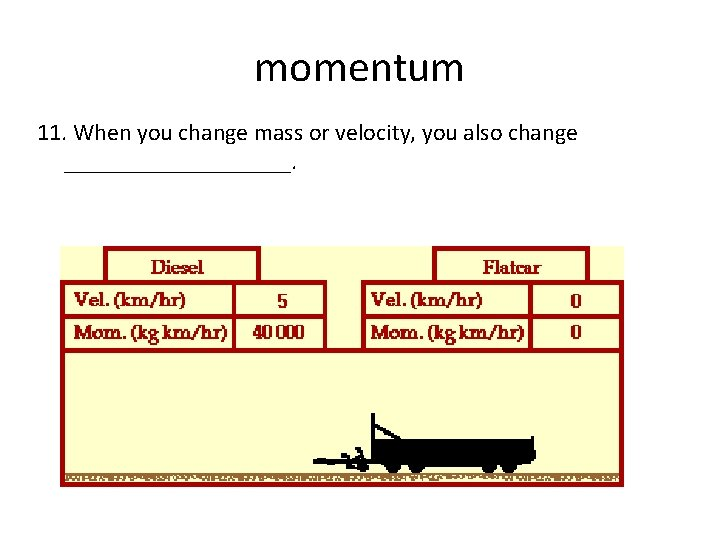momentum 11. When you change mass or velocity, you also change __________.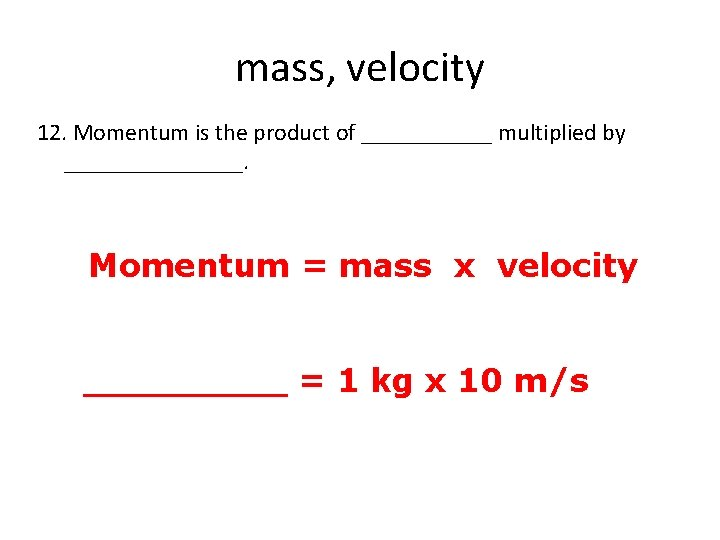mass, velocity 12. Momentum is the product of ______ multiplied by ________. Momentum = mass x velocity _____ = 1 kg x 10 m/skilogram, gram 13. Units of momentum are equal to units of mass times unit s of velocity, most often __________ meters per second (kg m/s) or ________ meters per second (g m/s). Bucket filled with sand is more difficult to be moved. It's also more difficult to be stopped from swinging.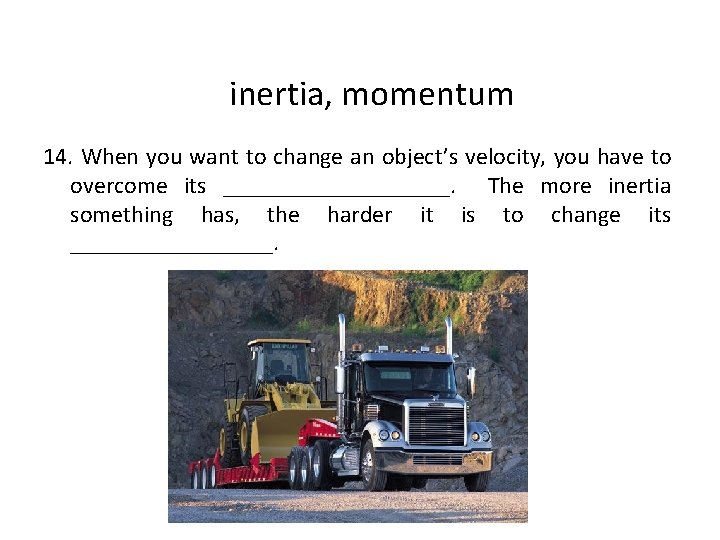inertia, momentum 14. When you want to change an object’s velocity, you have to overcome its __________. The more inertia something has, the harder it is to change its _________.